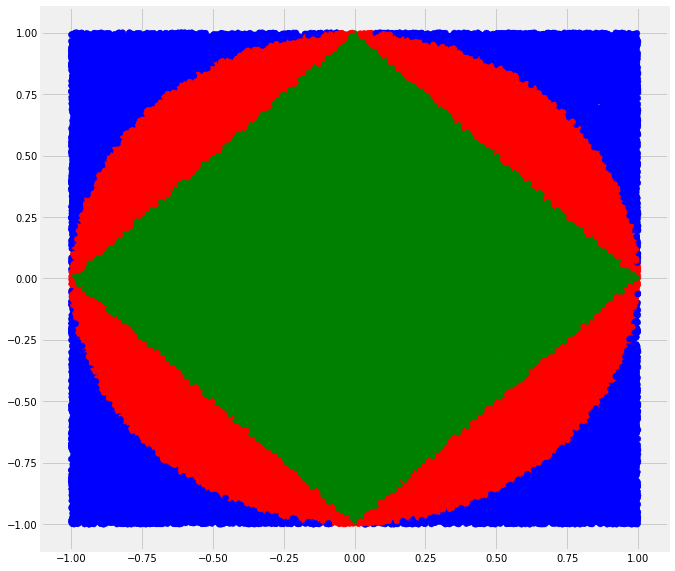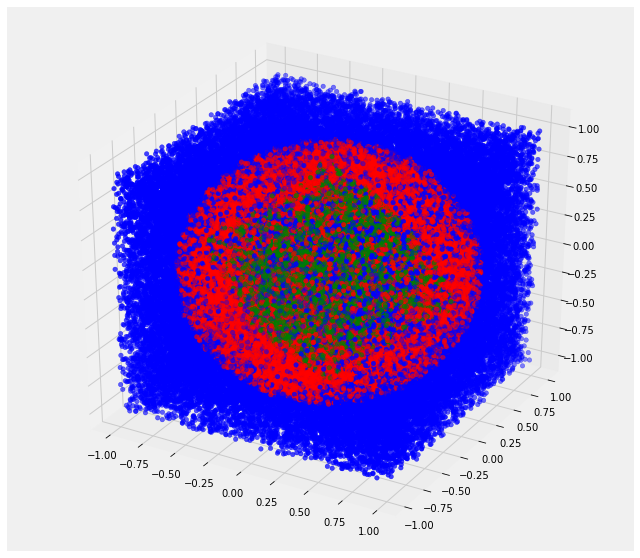# Building Intuition with Norm Balls in Hyperdimensions

Posted 2019-02-01

In :
from mpl_toolkits.mplot3d import Axes3D
import matplotlib.pyplot as plt
import numpy as np

%matplotlib inline
plt.style.use('fivethirtyeight')


## Building Intuition for Norm Balls in Hyperdimensions¶

• While we can visualize what happens in 2D and 3D, oftentimes our intuitions can break down in high dimensional space
• We 'throw a bunch of darts into space' to 'see' what happens in high dimensional space even if we cannot visualize it

### Properties of norms, though L1, L2, ... L infinity are calculated differently¶

• $ρ(x)>=0$ and $ρ(x)=0 \iff x=0$
• $ρ(αx) = |α|ρ(x)$
• 2 vectors $x$ and $y$, $ρ(x+y) \le ρ(x) + ρ(y)$
In :
# GLOBAL VARS
SHOW_N = 10
TRIALS = 100000

In :
class DartSimulation():
"""A simulation where we generate many random points and group the points
based on where they fall relative to the different norms.  With many trials
we can calculate relative sizes of different norm balls in high dimensional
space.

Parameters
----------
n : int
Number of times to 'throw' the dart
dim : int
Number of dimensions [default: 2]
magnitude : int
Max magnitude from origin [default: 1]

Attributes
----------
pts : ndarray, float
random n x dim matrix centered about origin +/- magnitude/2

L1_norm : ndarray, float
L1 norm of pts

L2_norm : ndarray, float
L2 norm of pts

Linf_norm : ndarray, float
L infinity norm of points

pt_colors : array, string
colors for pt
"""
def __init__(self, n, dim=2, magnitude=1):
self.pts = self.throw_darts(n, dim, magnitude)

def throw_darts(self, n, dim, magnitude):
return (2*magnitude) * np.random.random([n, dim]) - magnitude

@property
def L1_norm(self):
"""Sum of |xi| for i from 1 to n."""
self._L1_norm = abs(self.pts).sum(axis=1)
return self._L1_norm

@property
def L2_norm(self):
"""(x1^2 + x2^2 + ...xi^2)^(1/2) for i from 1 to n."""
self._L2_norm = (self.pts * self.pts).sum(axis=1)**(1/2)
return self._L2_norm

@property
def Linf_norm(self):
"""Max{|x1|, |x2|, ..., |xi|} for i from 1 to n."""
self._Linf_norm = abs(self.pts).max(axis=1)
return self._Linf_norm

@property
def pt_colors(self):
# Check calculated norms against boundary of norm balls L1, L2

# Map different colors for inside different norm balls
color_map = {(False, False): 'b',
(False, True): 'r',
(True, True): 'g'}
self._pt_colors = np.array([color_map[pt] for pt in norm_mask])
return self._pt_colors

def plot_pts_2d(self):
"""Plot the color coded points in 2D"""
plt.figure(figsize=(10,10))
plt.scatter(self.pts[:, 0], self.pts[:, 1], c=self.pt_colors)

def plot_pts_3d(self):
"""Plot the color coded points in 3D"""
fig = plt.figure(figsize=(10,10))
ax.scatter(self.pts[:, 0],
self.pts[:, 1],
self.pts[:, 2],
c=self.pt_colors)

In :
def norm_ratios(pt_colors):
"""Calculate ratios of L1, L2, L infinity norm balls.

Parameters
----------
pt_colors : array, str
color of pts

Returns
-------
Float ratio of L1 / L infinity, ratio of L2 / L infinity
"""
L1_pts = len(pt_colors[pt_colors == 'g'])
L2_pts = len(pt_colors[pt_colors == 'r'])
Linf_pts = len(pt_colors[pt_colors == 'b'])
total_pts = L1_pts + L2_pts + Linf_pts
return L1_pts / total_pts, (L1_pts + L2_pts) / total_pts

In :
# Start the simulation by 'throwing a bunch of darts'
r2 = DartSimulation(TRIALS)
r2.pts[:SHOW_N]

Out:
array([[ 0.307593  ,  0.74479625],
[-0.74942285, -0.55153295],
[-0.88005674,  0.38066115],
[ 0.84426139, -0.64569835],
[-0.41294356, -0.91373221],
[-0.5482814 , -0.43180403],
[-0.02916798, -0.02511579],
[-0.68285746, -0.30136359],
[ 0.14696013, -0.0958312 ],
[-0.62032417,  0.87630596]])
In :
# Calculate L1, L2, L infinity for the set of points
r2.L1_norm[:SHOW_N], r2.L2_norm[:SHOW_N], r2.Linf_norm[:SHOW_N]

Out:
(array([1.05238926, 1.3009558 , 1.26071788, 1.48995974, 1.32667577,
0.98008543, 0.05428378, 0.98422105, 0.24279134, 1.49663013]),
array([0.8058132 , 0.93049621, 0.95885493, 1.06287518, 1.00271079,
0.69790201, 0.03849122, 0.74640091, 0.17544486, 1.07364529]),
array([0.74479625, 0.74942285, 0.88005674, 0.84426139, 0.91373221,
0.5482814 , 0.02916798, 0.68285746, 0.14696013, 0.87630596]))

## L1, L2, L infinity norm balls in 2D¶

In :
# Green if inside L1 norm ball, red if > L1 and < L2, blue if > L2
r2.pt_colors[:SHOW_N]

Out:
array(['r', 'r', 'r', 'b', 'b', 'g', 'g', 'g', 'g', 'b'], dtype='<U1')
In :
r2.plot_pts_2d()In :
# Ratio of L1 / L infinity, L2 / L infinity
norm_ratios(r2.pt_colors)

Out:
(0.49839, 0.78464)
• L1 norm ball (looks like square) is 1/2 the area of L infinity so ratio of L1 / L infinity = 0.5
• L2 norm ball is area of a circle = $\pi r^2$ and since $r=1$ thus L2 / L infinity ~= 0.785

The 'dart throwing' results are inline with the calculated ones.

## L1, L2, L infinity norm balls in 3D¶

In :
r3 = DartSimulation(TRIALS, 3)
r3.plot_pts_3d()In :
norm_ratios(r3.pt_colors)

Out:
(0.16682, 0.52593)
• L1 norm ball (looks like 8 sided die or octahedron) is volume ${\frac {\sqrt2} 3 } edge^3$ so L1 / L infinity = $\frac {{\frac {\sqrt2} 3 } {\sqrt2}^3} {2^3}$ so ~= 0.167
• L2 norm ball is volume $\frac 4 3 \pi$ so L2 / L infinity = $\frac{\frac 4 3 \pi} {2^3}$ which ~= 0.52

The 'dart throwing' results are inline with the calculated ones.

## Ratios of L1 / L infinity and L2 / L infinity in high dimensions (2 -> 10D)¶

Note how as dimensions get higher and higher L1 and L2 are so much smaller than L infinity!

In :
for d in range(2, 11):
x = DartSimulation(TRIALS, d)
print(norm_ratios(x.pt_colors))

(0.50019, 0.78569)
(0.16863, 0.52546)
(0.04118, 0.30796)
(0.00859, 0.16456)
(0.0016, 0.07968)
(0.00021, 0.03691)
(0.0, 0.01561)
(0.0, 0.00646)
(0.0, 0.00255)